Скачать презентацию Modern Atomic Theory 1 2 Electromagnetic radiation

6914e628c1f675940c4580db8105a135.ppt

• Количество слайдов: 63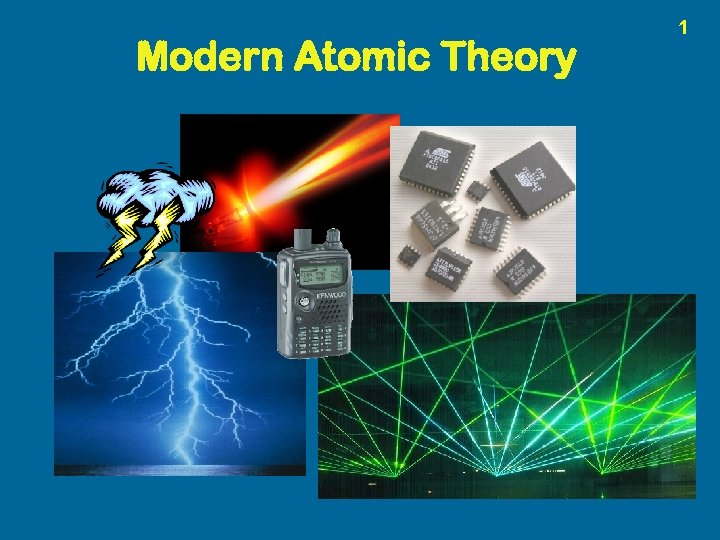Modern Atomic Theory 1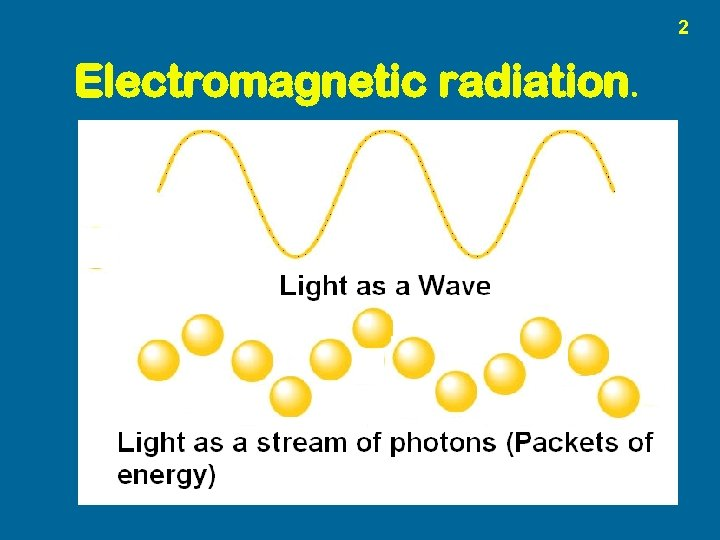2 Electromagnetic radiation.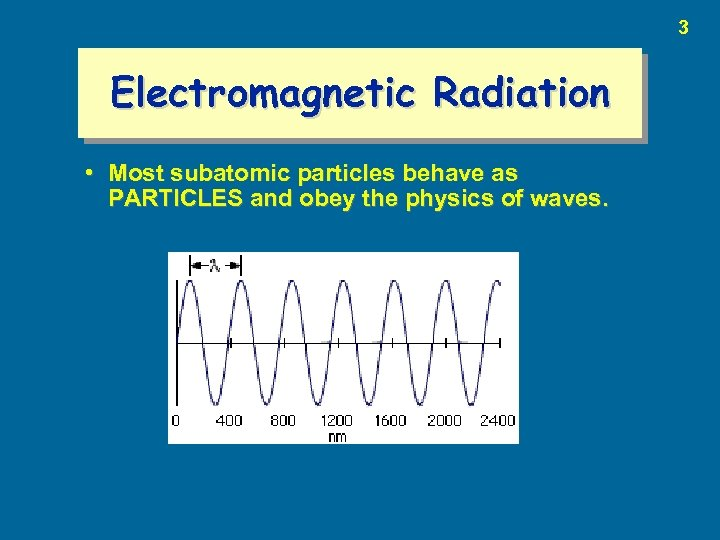3 Electromagnetic Radiation • Most subatomic particles behave as PARTICLES and obey the physics of waves.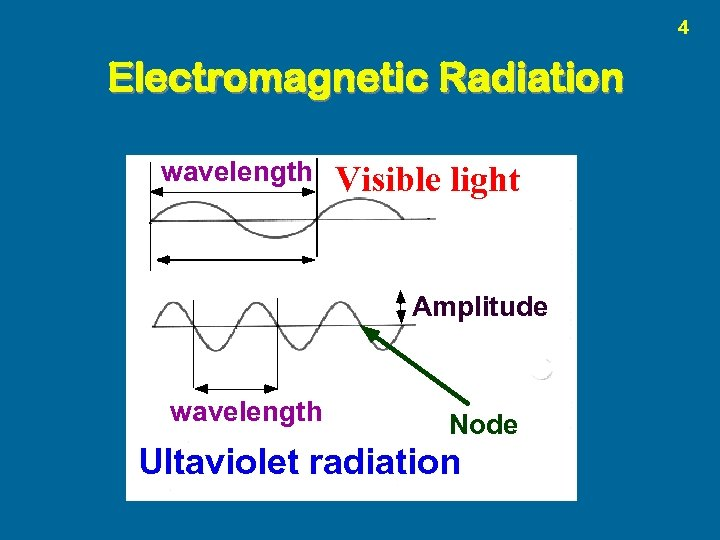4 Electromagnetic Radiation wavelength Visible light Amplitude wavelength Node Ultaviolet radiation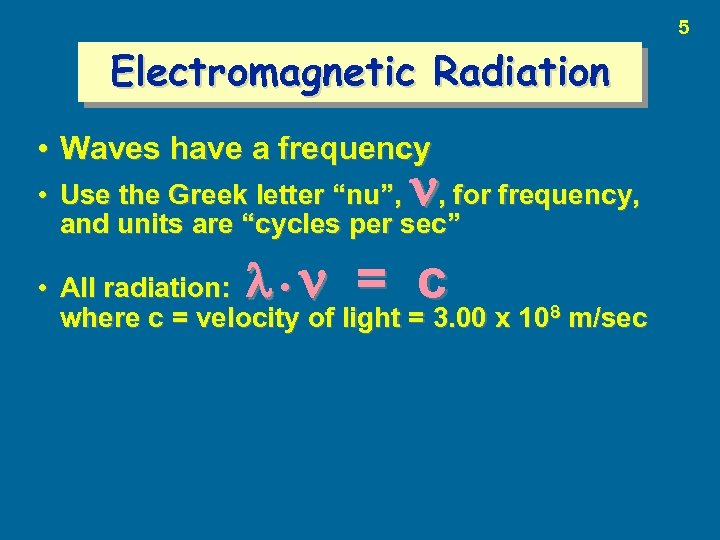5 Electromagnetic Radiation • Waves have a frequency • Use the Greek letter “nu”, , for frequency, and units are “cycles per sec” = c • All radiation: • where c = velocity of light = 3. 00 x 108 m/sec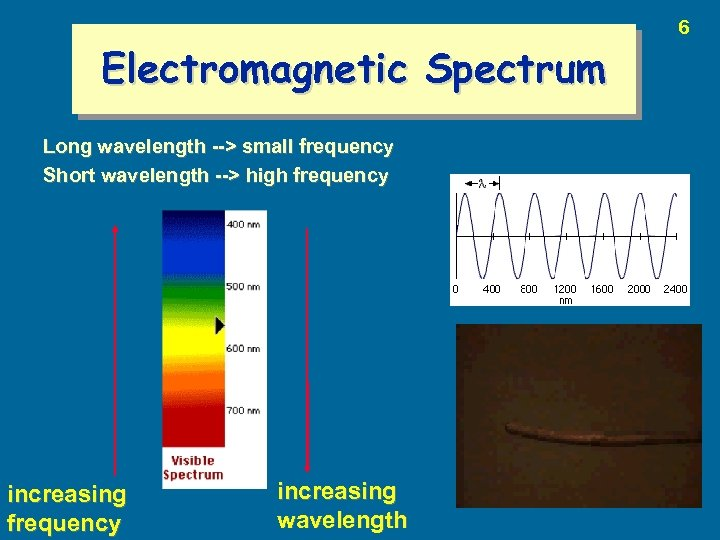Electromagnetic Spectrum Long wavelength --> small frequency Short wavelength --> high frequency increasing wavelength 6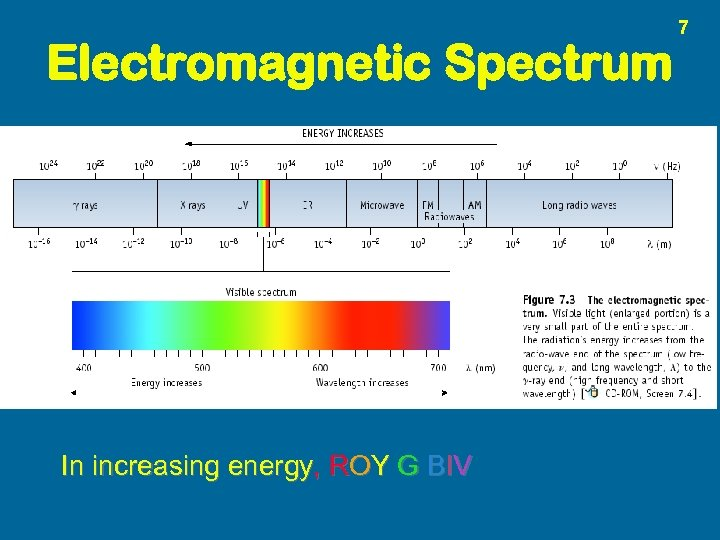Electromagnetic Spectrum In increasing energy, ROY G BIV 7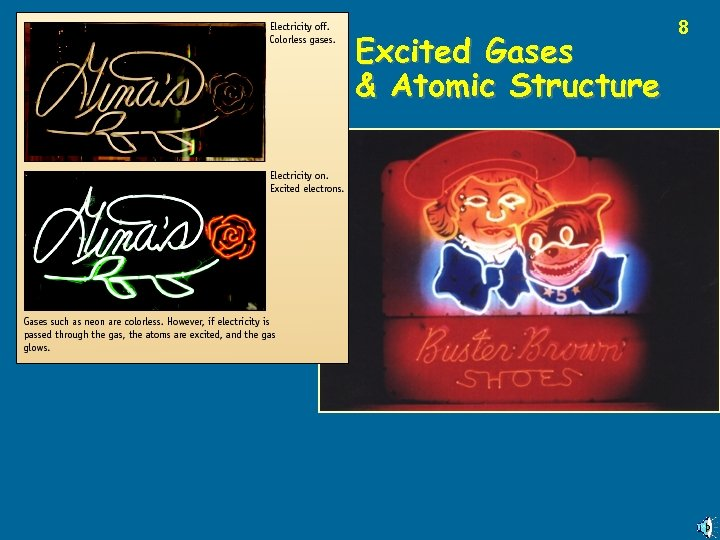Excited Gases & Atomic Structure 8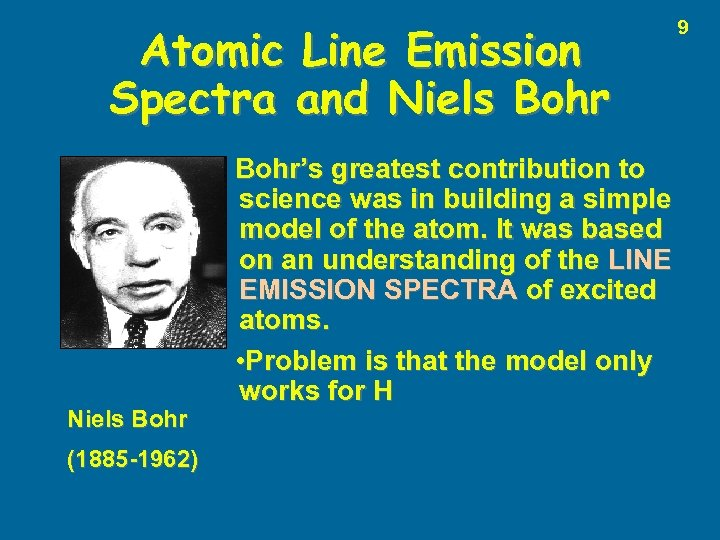Atomic Line Emission Spectra and Niels Bohr (1885 -1962) Bohr’s greatest contribution to science was in building a simple model of the atom. It was based on an understanding of the LINE EMISSION SPECTRA of excited atoms. • Problem is that the model only works for H 9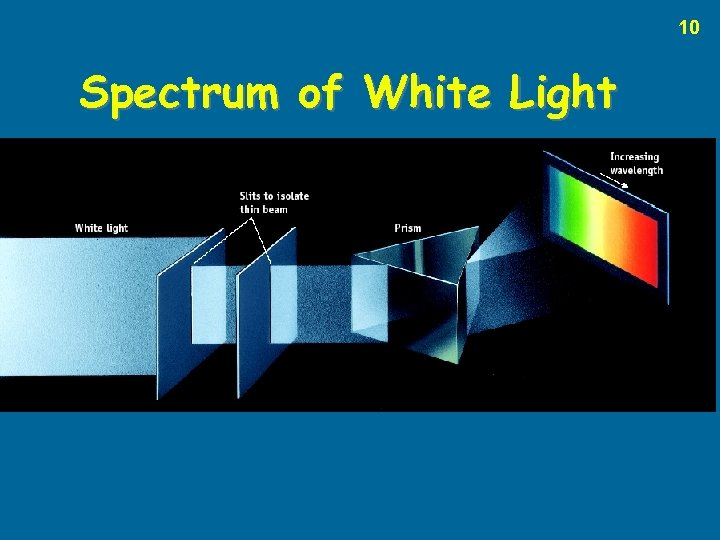10 Spectrum of White Light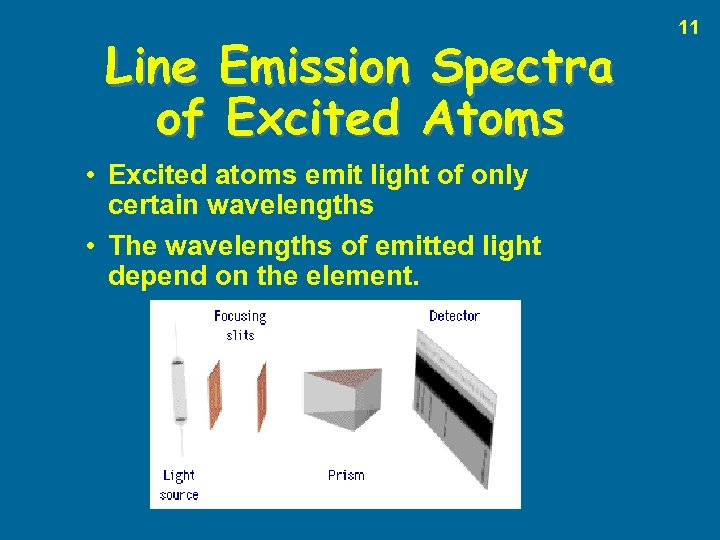Line Emission Spectra of Excited Atoms • Excited atoms emit light of only certain wavelengths • The wavelengths of emitted light depend on the element. 11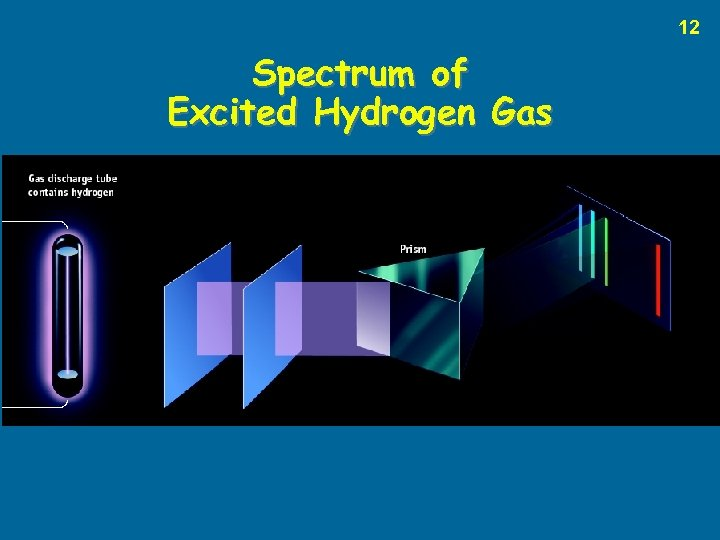12 Spectrum of Excited Hydrogen Gas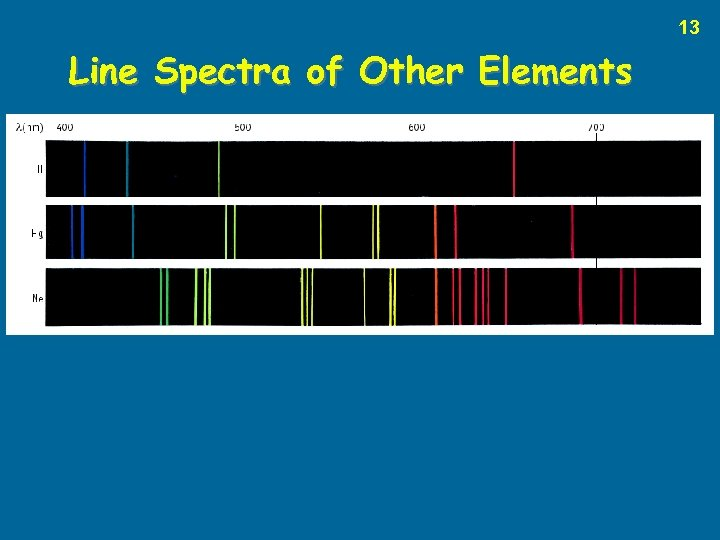13 Line Spectra of Other Elements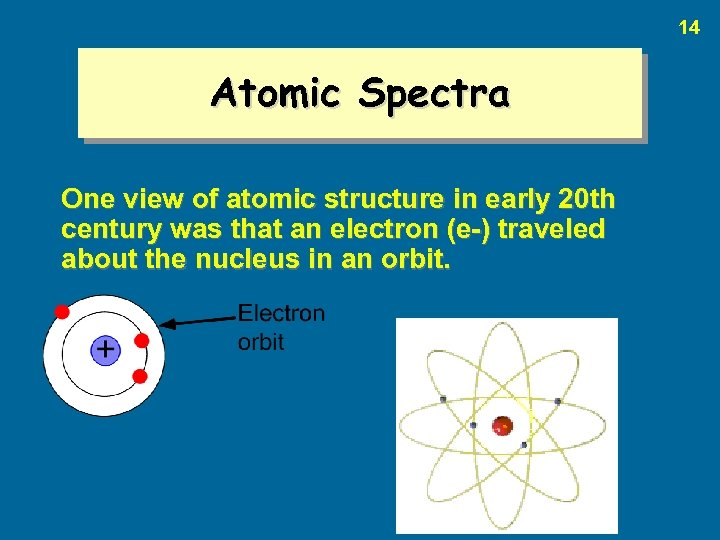14 Atomic Spectra One view of atomic structure in early 20 th century was that an electron (e-) traveled about the nucleus in an orbit.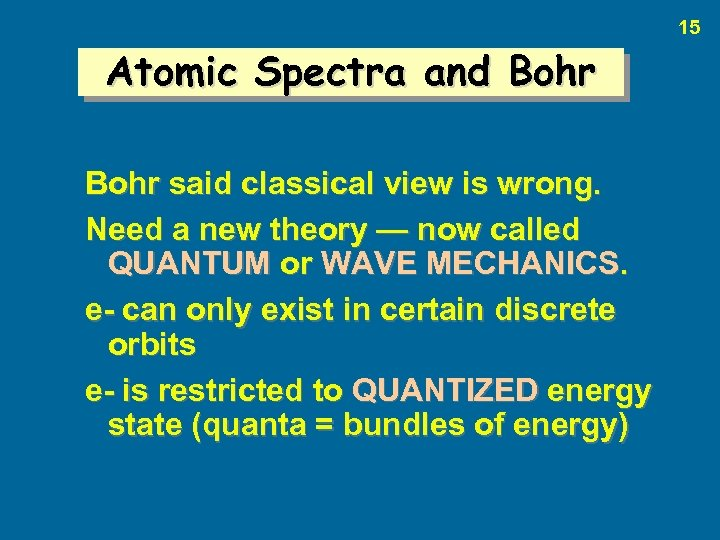15 Atomic Spectra and Bohr said classical view is wrong. Need a new theory — now called QUANTUM or WAVE MECHANICS. e- can only exist in certain discrete orbits e- is restricted to QUANTIZED energy state (quanta = bundles of energy)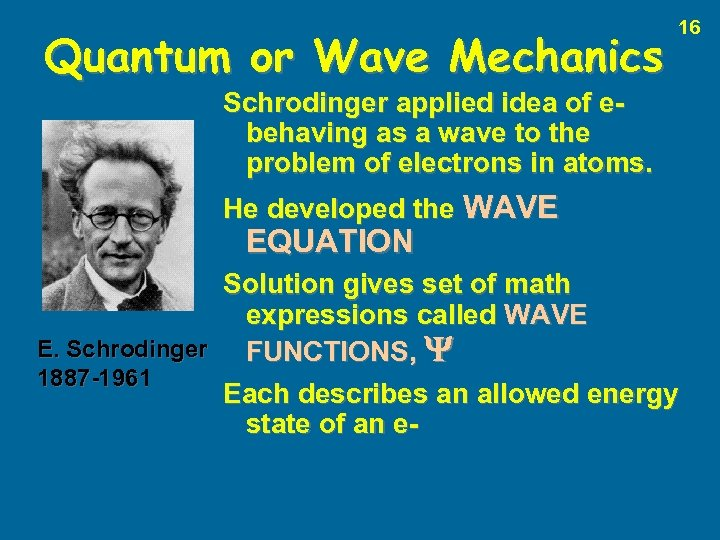Quantum or Wave Mechanics 16 Schrodinger applied idea of ebehaving as a wave to the problem of electrons in atoms. He developed the WAVE EQUATION Solution gives set of math expressions called WAVE E. Schrodinger FUNCTIONS, 1887 -1961 Each describes an allowed energy state of an e-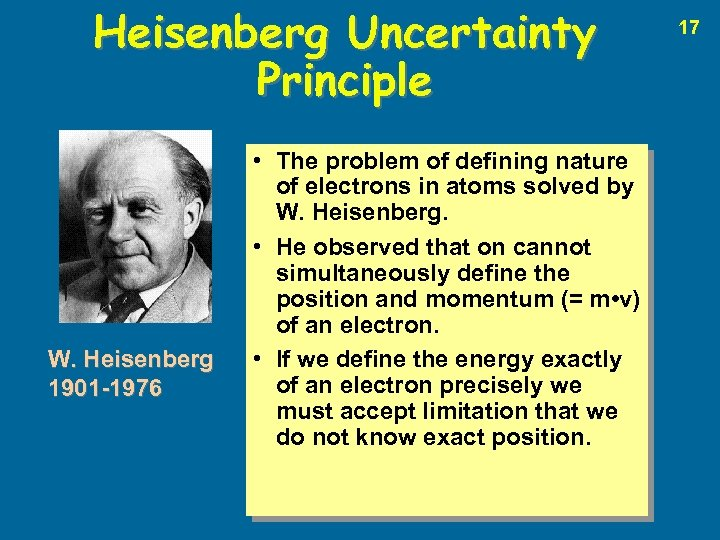Heisenberg Uncertainty Principle W. Heisenberg 1901 -1976 • The problem of defining nature of electrons in atoms solved by W. Heisenberg. • He observed that on cannot simultaneously define the position and momentum (= m • v) of an electron. • If we define the energy exactly of an electron precisely we must accept limitation that we do not know exact position. 17Arrangement of Electrons in Atoms Electrons in atoms are arranged as LEVELS (n) SUBLEVELS (l) ORBITALS (ml) 18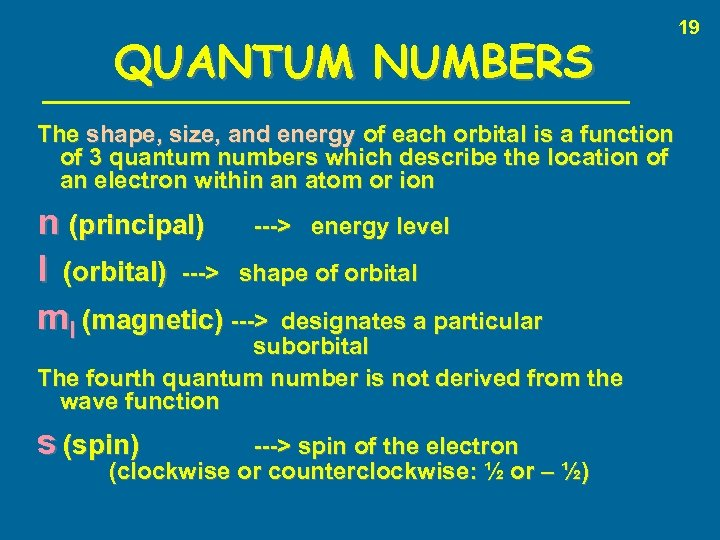QUANTUM NUMBERS The shape, size, and energy of each orbital is a function of 3 quantum numbers which describe the location of an electron within an atom or ion n (principal) ---> energy level l (orbital) ---> shape of orbital ml (magnetic) ---> designates a particular suborbital The fourth quantum number is not derived from the wave function s (spin) ---> spin of the electron (clockwise or counterclockwise: ½ or – ½) 19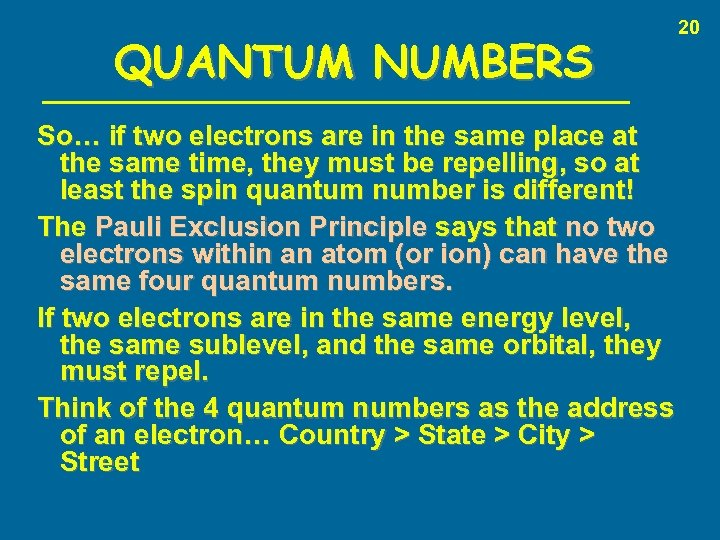QUANTUM NUMBERS So… if two electrons are in the same place at the same time, they must be repelling, so at least the spin quantum number is different! The Pauli Exclusion Principle says that no two electrons within an atom (or ion) can have the same four quantum numbers. If two electrons are in the same energy level, the same sublevel, and the same orbital, they must repel. Think of the 4 quantum numbers as the address of an electron… Country > State > City > Street 20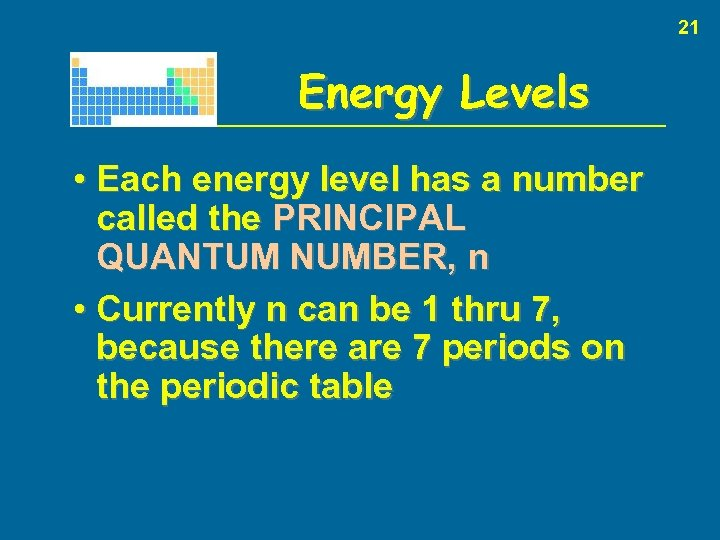21 Energy Levels • Each energy level has a number called the PRINCIPAL QUANTUM NUMBER, n • Currently n can be 1 thru 7, because there are 7 periods on the periodic table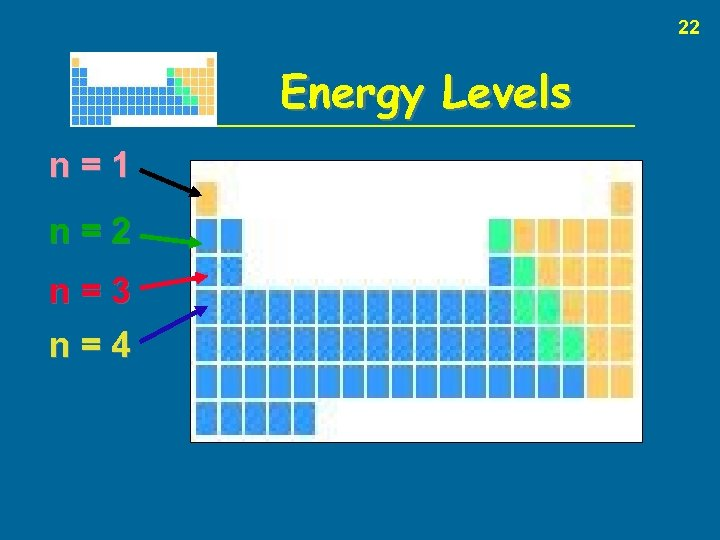22 Energy Levels n=1 n=2 n=3 n=4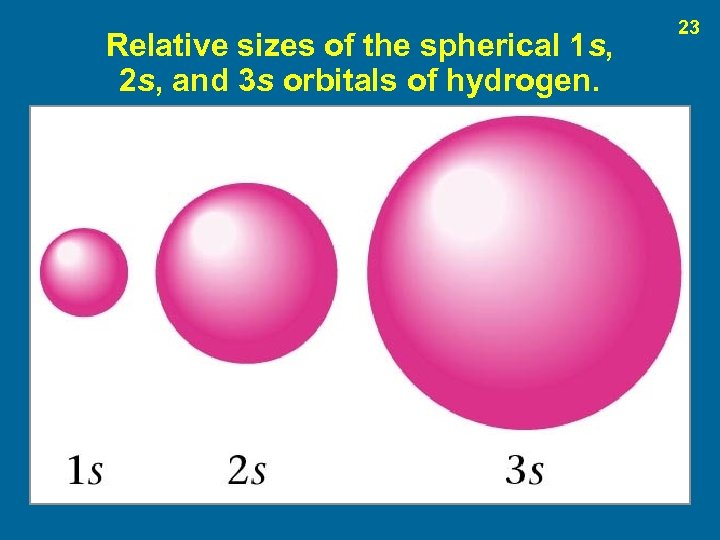Relative sizes of the spherical 1 s, 2 s, and 3 s orbitals of hydrogen. 23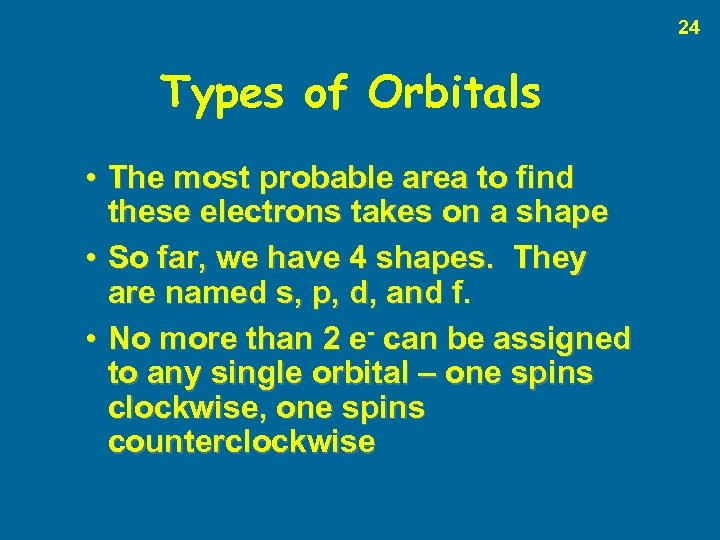24 Types of Orbitals • The most probable area to find these electrons takes on a shape • So far, we have 4 shapes. They are named s, p, d, and f. • No more than 2 e- can be assigned to any single orbital – one spins clockwise, one spins counterclockwise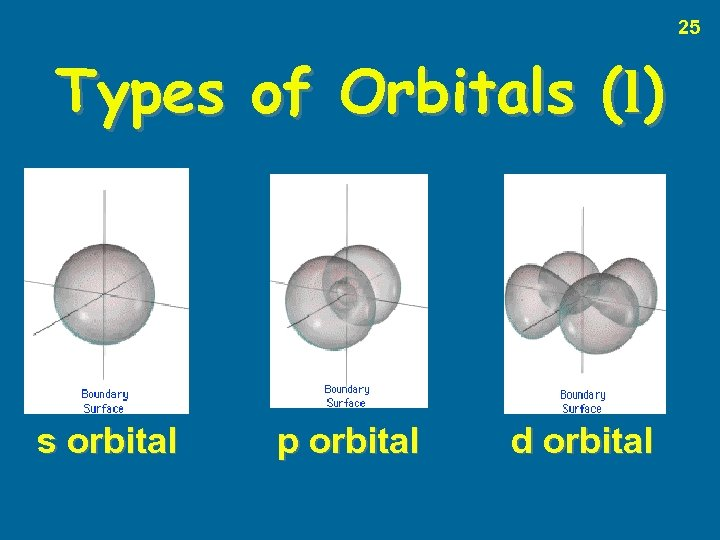25 Types of Orbitals (l) s orbital p orbital d orbital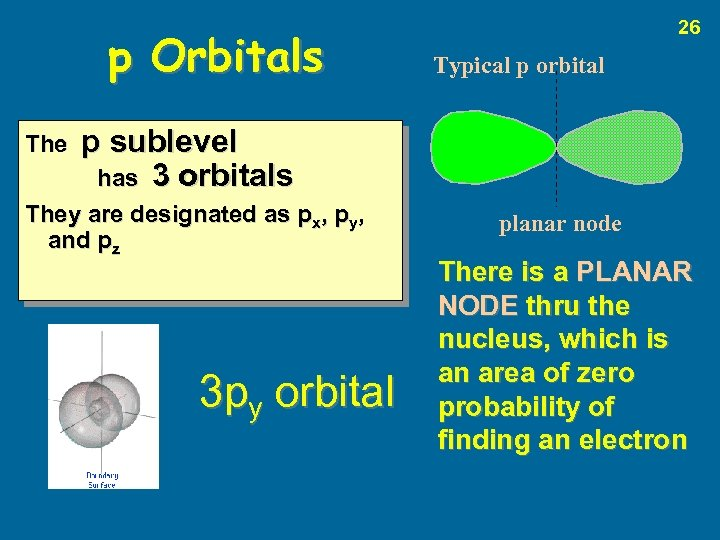p Orbitals The 26 Typical p orbital p sublevel has 3 orbitals They are designated as px, py, and pz 3 py orbital planar node There is a PLANAR NODE thru the nucleus, which is an area of zero probability of finding an electron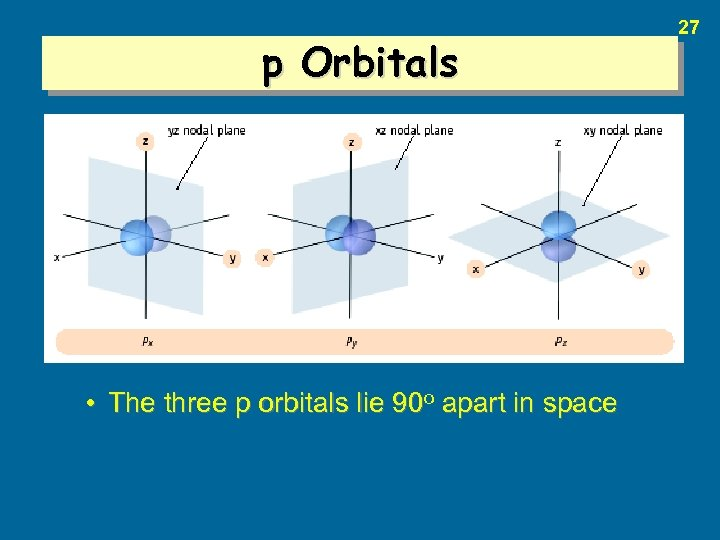p Orbitals • The three p orbitals lie 90 o apart in space 27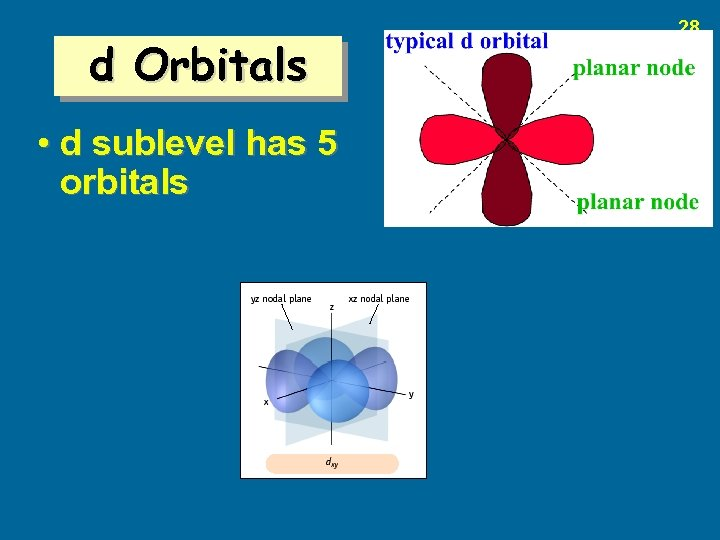d Orbitals • d sublevel has 5 orbitals 28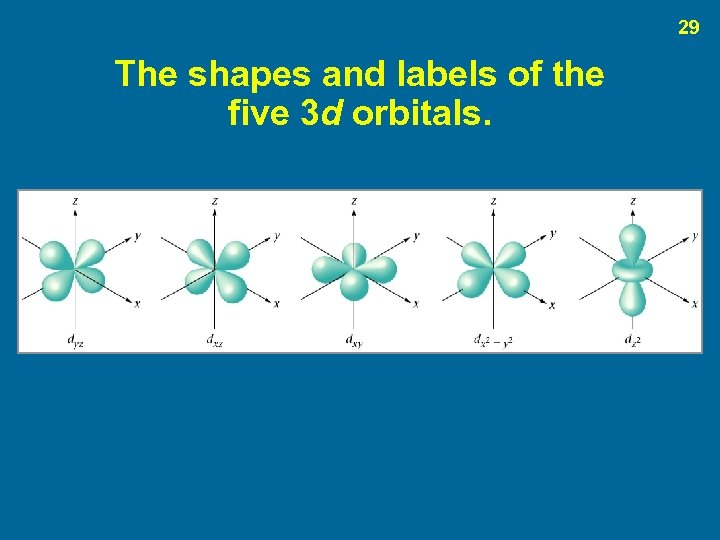29 The shapes and labels of the five 3 d orbitals.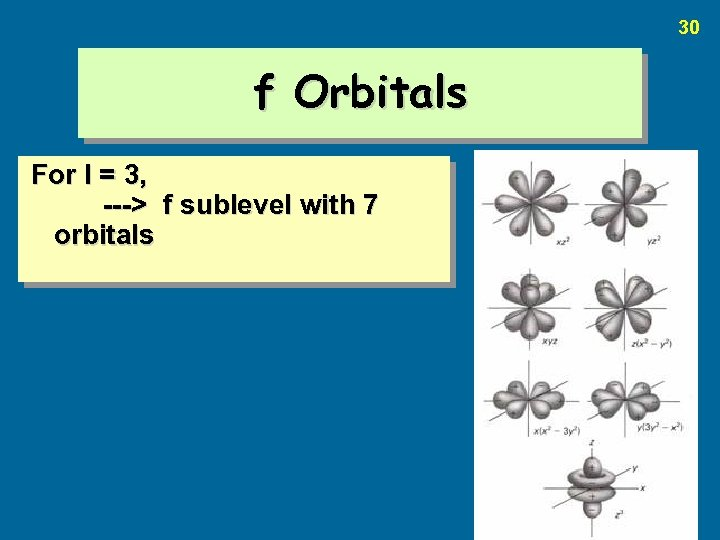30 f Orbitals For l = 3, ---> f sublevel with 7 orbitals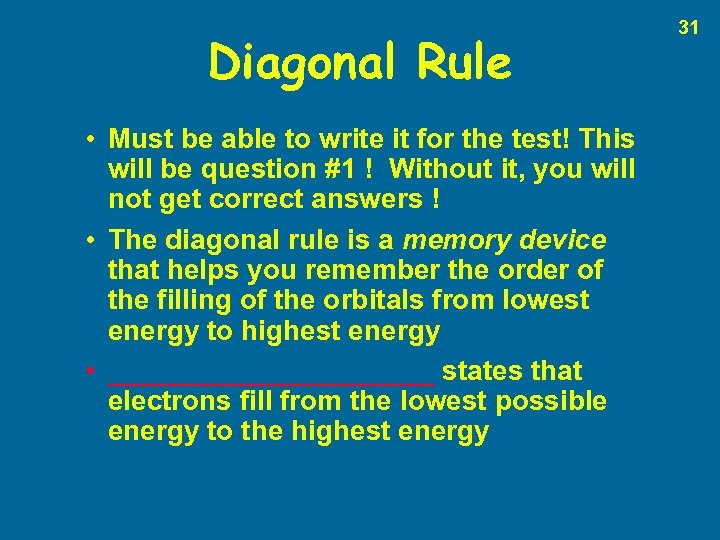Diagonal Rule • Must be able to write it for the test! This will be question #1 ! Without it, you will not get correct answers ! • The diagonal rule is a memory device that helps you remember the order of the filling of the orbitals from lowest energy to highest energy • ___________ states that electrons fill from the lowest possible energy to the highest energy 31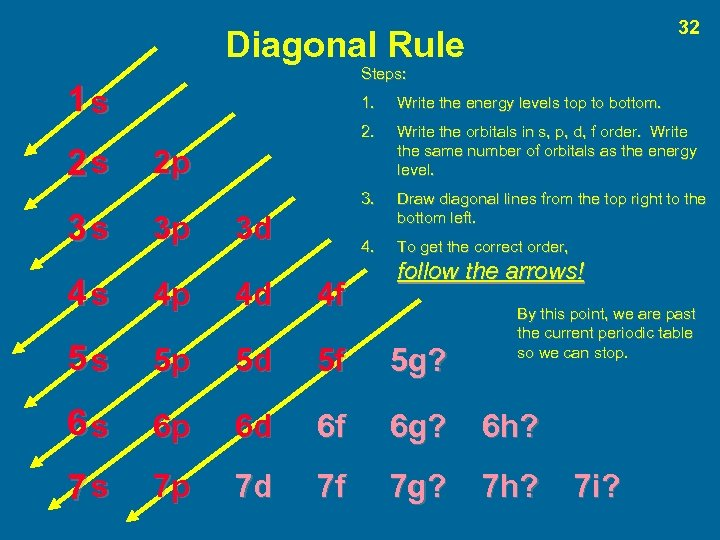32 Diagonal Rule Steps: 1 s 3 s Write the energy levels top to bottom. 2 s 1. Write the orbitals in s, p, d, f order. Write the same number of orbitals as the energy level. 3. Draw diagonal lines from the top right to the bottom left. 4. To get the correct order, 2 p 3 p 3 d follow the arrows! 4 s 4 p 4 d 4 f 5 s 5 p 5 d 5 f 5 g? 6 s 6 p 6 d 6 f 6 g? 6 h? 7 s 7 p 7 d 7 f 7 g? 7 h? By this point, we are past the current periodic table so we can stop. 7 i?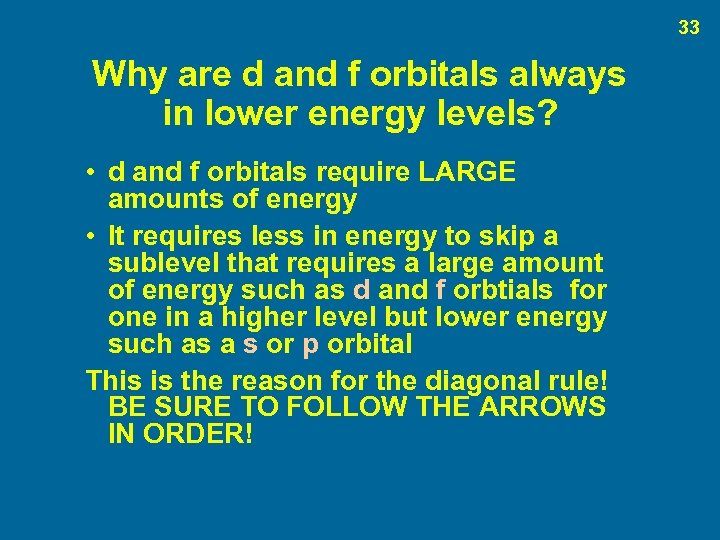33 Why are d and f orbitals always in lower energy levels? • d and f orbitals require LARGE amounts of energy • It requires less in energy to skip a sublevel that requires a large amount of energy such as d and f orbtials for one in a higher level but lower energy such as a s or p orbital This is the reason for the diagonal rule! BE SURE TO FOLLOW THE ARROWS IN ORDER!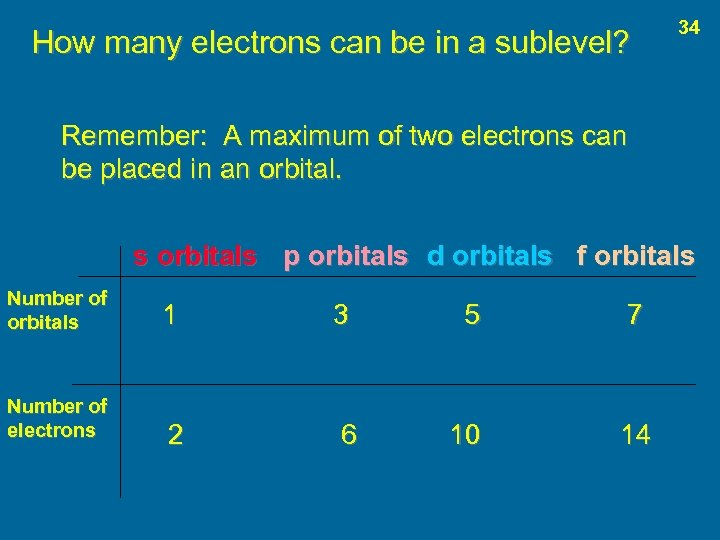How many electrons can be in a sublevel? 34 Remember: A maximum of two electrons can be placed in an orbital. s orbitals p orbitals d orbitals f orbitals Number of orbitals 1 3 5 7 Number of electrons 2 6 10 14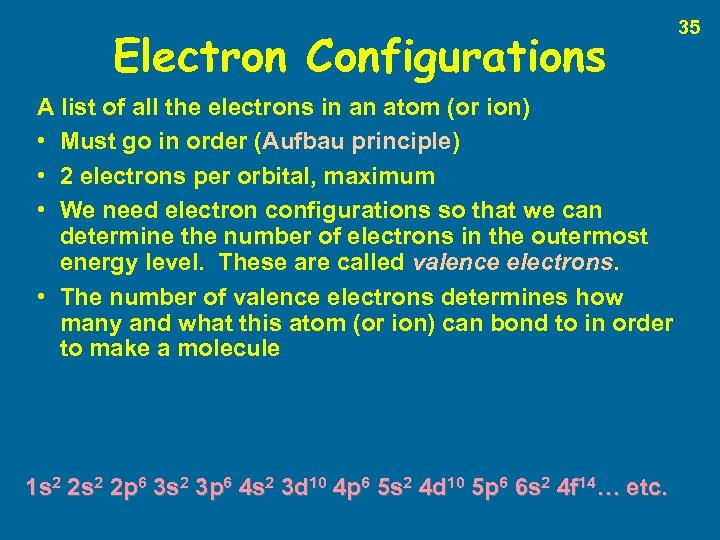Electron Configurations A list of all the electrons in an atom (or ion) • Must go in order (Aufbau principle) • 2 electrons per orbital, maximum • We need electron configurations so that we can determine the number of electrons in the outermost energy level. These are called valence electrons. • The number of valence electrons determines how many and what this atom (or ion) can bond to in order to make a molecule 1 s 2 2 p 6 3 s 2 3 p 6 4 s 2 3 d 10 4 p 6 5 s 2 4 d 10 5 p 6 6 s 2 4 f 14… etc. 35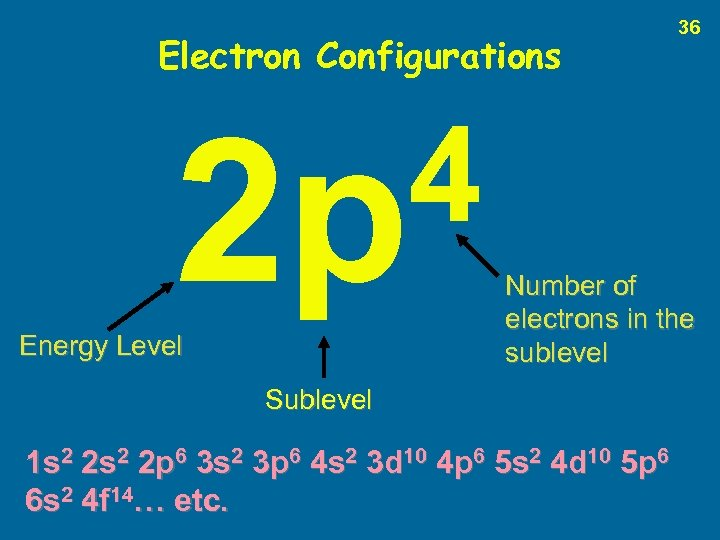Electron Configurations 4 2 p Energy Level 36 Number of electrons in the sublevel Sublevel 1 s 2 2 p 6 3 s 2 3 p 6 4 s 2 3 d 10 4 p 6 5 s 2 4 d 10 5 p 6 6 s 2 4 f 14… etc.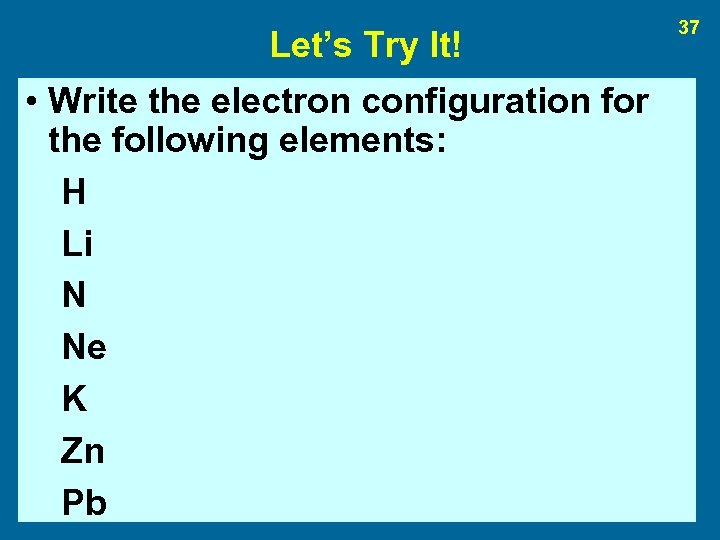Let’s Try It! • Write the electron configuration for the following elements: H Li N Ne K Zn Pb 37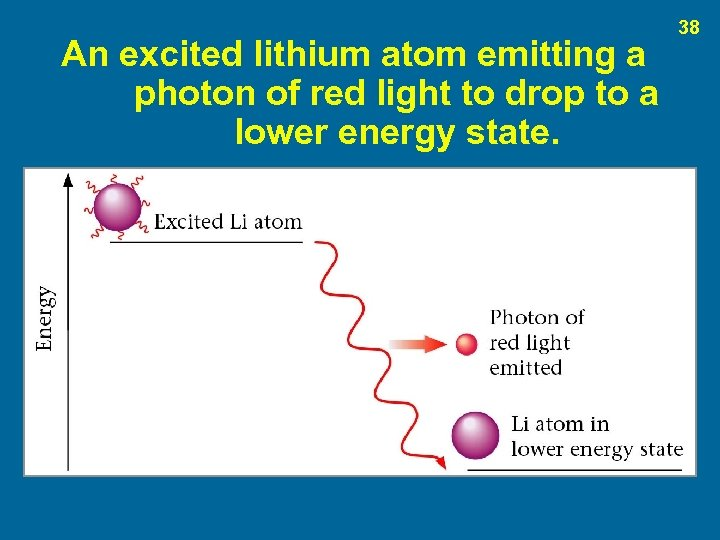An excited lithium atom emitting a photon of red light to drop to a lower energy state. 38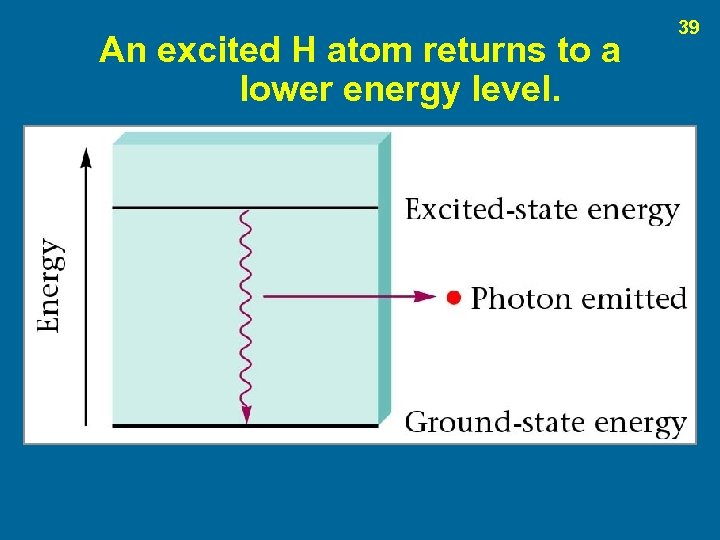An excited H atom returns to a lower energy level. 39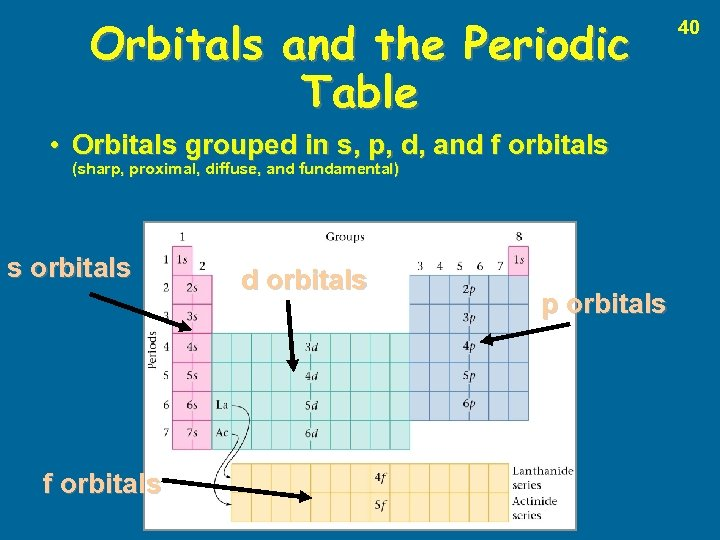Orbitals and the Periodic Table • Orbitals grouped in s, p, d, and f orbitals (sharp, proximal, diffuse, and fundamental) s orbitals f orbitals d orbitals p orbitals 40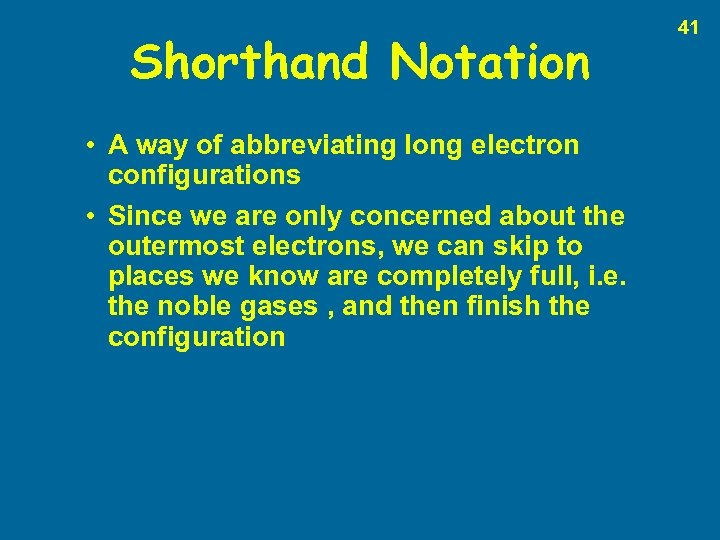Shorthand Notation • A way of abbreviating long electron configurations • Since we are only concerned about the outermost electrons, we can skip to places we know are completely full, i. e. the noble gases , and then finish the configuration 41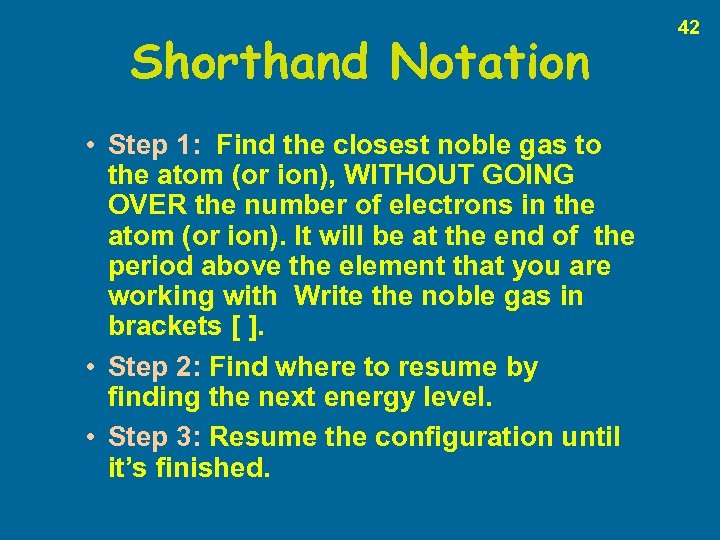Shorthand Notation • Step 1: Find the closest noble gas to the atom (or ion), WITHOUT GOING OVER the number of electrons in the atom (or ion). It will be at the end of the period above the element that you are working with Write the noble gas in brackets [ ]. • Step 2: Find where to resume by finding the next energy level. • Step 3: Resume the configuration until it’s finished. 42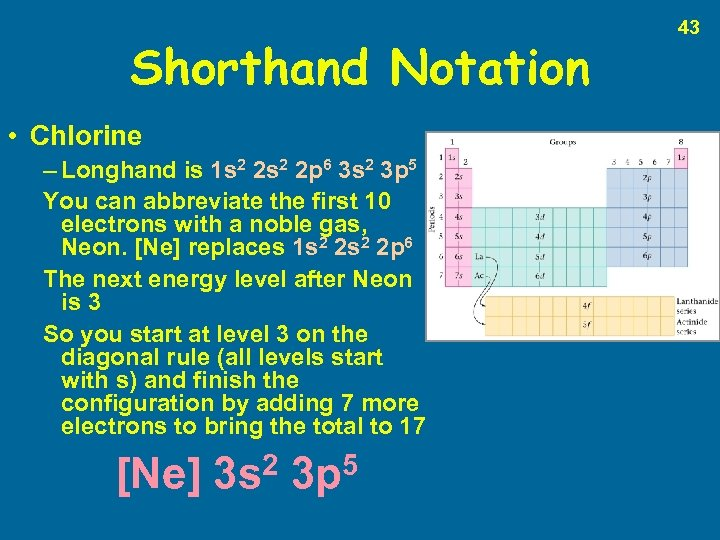Shorthand Notation • Chlorine – Longhand is 1 s 2 2 p 6 3 s 2 3 p 5 You can abbreviate the first 10 electrons with a noble gas, Neon. [Ne] replaces 1 s 2 2 p 6 The next energy level after Neon is 3 So you start at level 3 on the diagonal rule (all levels start with s) and finish the configuration by adding 7 more electrons to bring the total to 17 [Ne] 2 3 s 5 3 p 43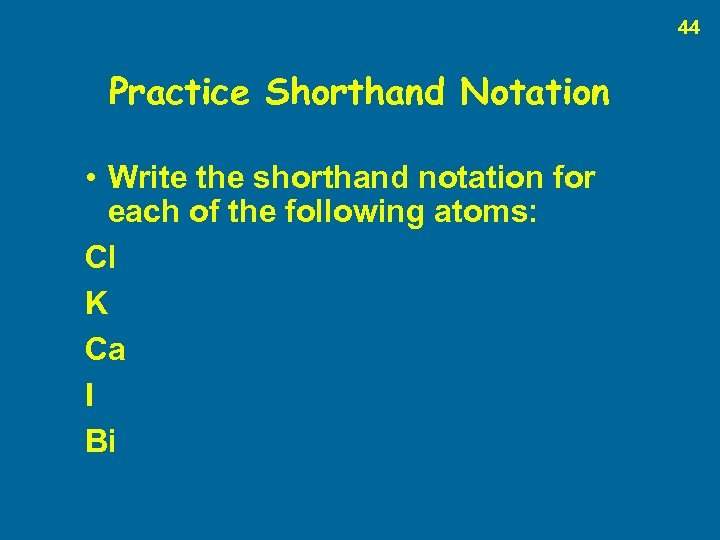44 Practice Shorthand Notation • Write the shorthand notation for each of the following atoms: Cl K Ca I Bi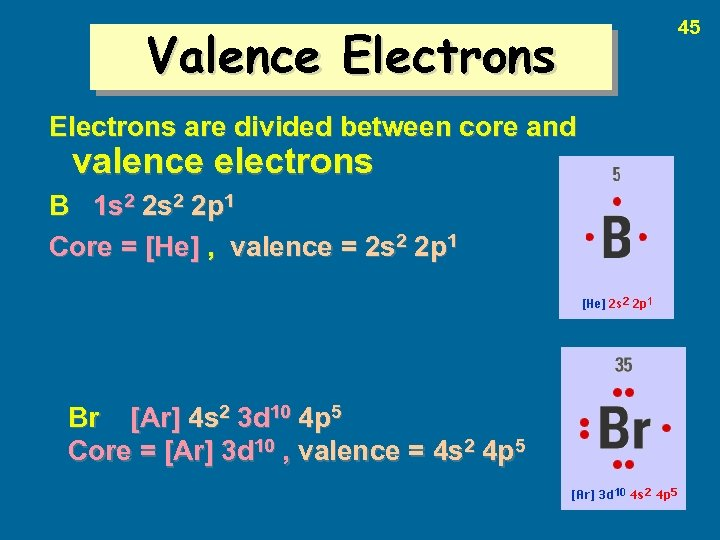Valence Electrons are divided between core and valence electrons B 1 s 2 2 p 1 Core = [He] , valence = 2 s 2 2 p 1 Br [Ar] 4 s 2 3 d 10 4 p 5 Core = [Ar] 3 d 10 , valence = 4 s 2 4 p 5 45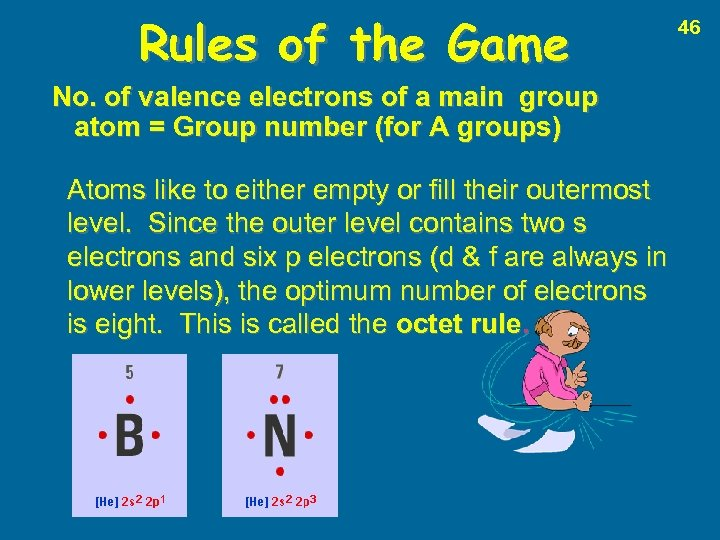Rules of the Game No. of valence electrons of a main group atom = Group number (for A groups) Atoms like to either empty or fill their outermost level. Since the outer level contains two s electrons and six p electrons (d & f are always in lower levels), the optimum number of electrons is eight. This is called the octet rule. 46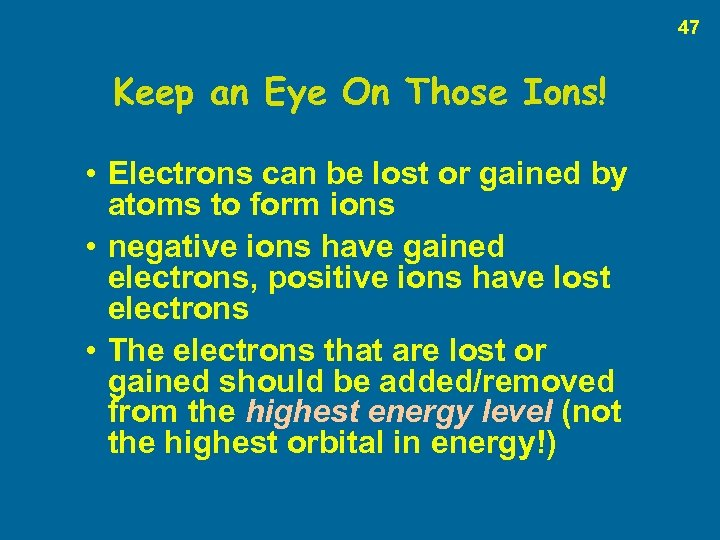47 Keep an Eye On Those Ions! • Electrons can be lost or gained by atoms to form ions • negative ions have gained electrons, positive ions have lost electrons • The electrons that are lost or gained should be added/removed from the highest energy level (not the highest orbital in energy!)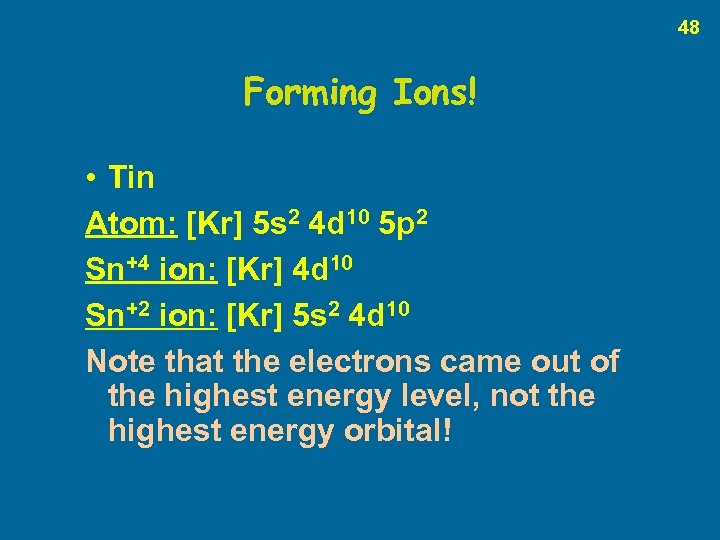48 Forming Ions! • Tin Atom: [Kr] 5 s 2 4 d 10 5 p 2 Sn+4 ion: [Kr] 4 d 10 Sn+2 ion: [Kr] 5 s 2 4 d 10 Note that the electrons came out of the highest energy level, not the highest energy orbital!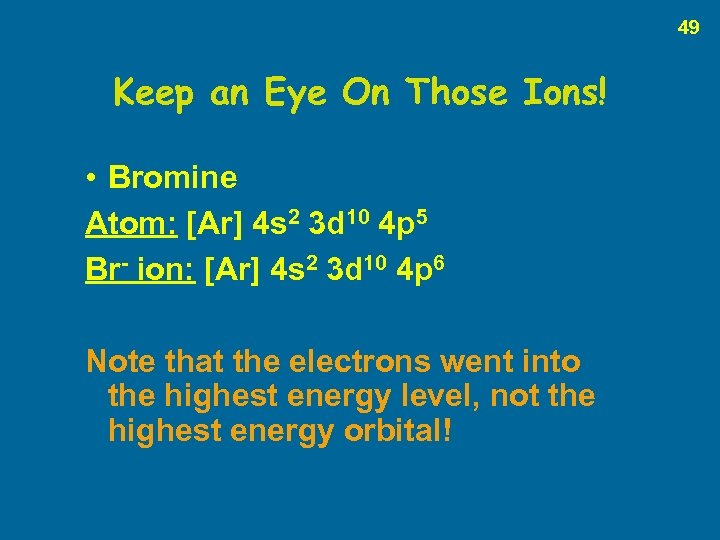49 Keep an Eye On Those Ions! • Bromine Atom: [Ar] 4 s 2 3 d 10 4 p 5 Br- ion: [Ar] 4 s 2 3 d 10 4 p 6 Note that the electrons went into the highest energy level, not the highest energy orbital!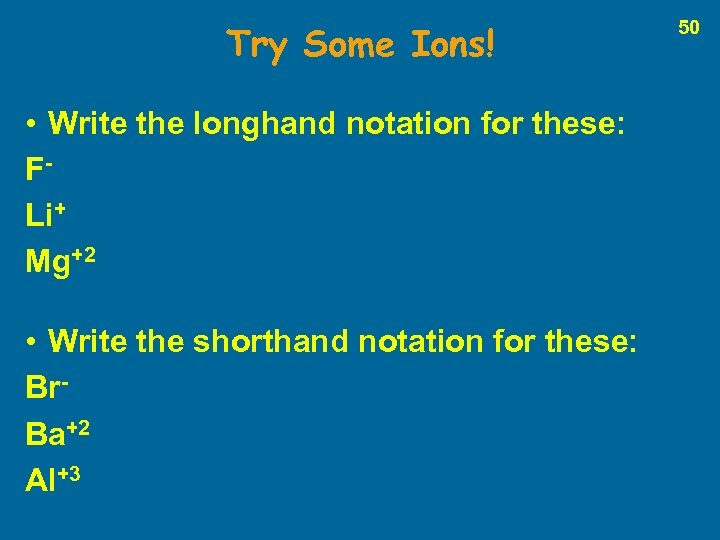Try Some Ions! • Write the longhand notation for these: FLi+ Mg+2 • Write the shorthand notation for these: Br. Ba+2 Al+3 50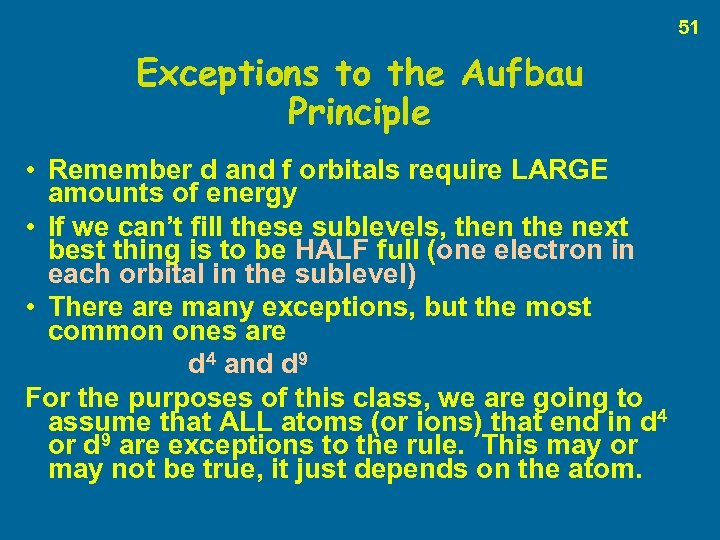51 Exceptions to the Aufbau Principle • Remember d and f orbitals require LARGE amounts of energy • If we can’t fill these sublevels, then the next best thing is to be HALF full (one electron in each orbital in the sublevel) • There are many exceptions, but the most common ones are d 4 and d 9 For the purposes of this class, we are going to assume that ALL atoms (or ions) that end in d 4 or d 9 are exceptions to the rule. This may or may not be true, it just depends on the atom.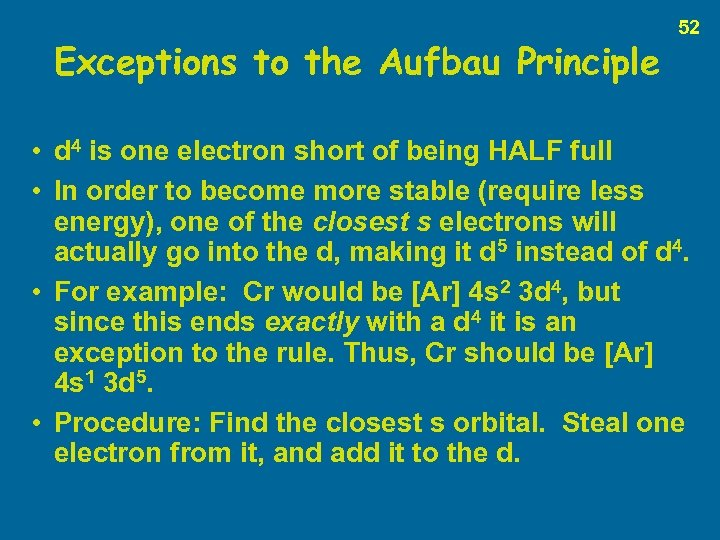Exceptions to the Aufbau Principle 52 • d 4 is one electron short of being HALF full • In order to become more stable (require less energy), one of the closest s electrons will actually go into the d, making it d 5 instead of d 4. • For example: Cr would be [Ar] 4 s 2 3 d 4, but since this ends exactly with a d 4 it is an exception to the rule. Thus, Cr should be [Ar] 4 s 1 3 d 5. • Procedure: Find the closest s orbital. Steal one electron from it, and add it to the d.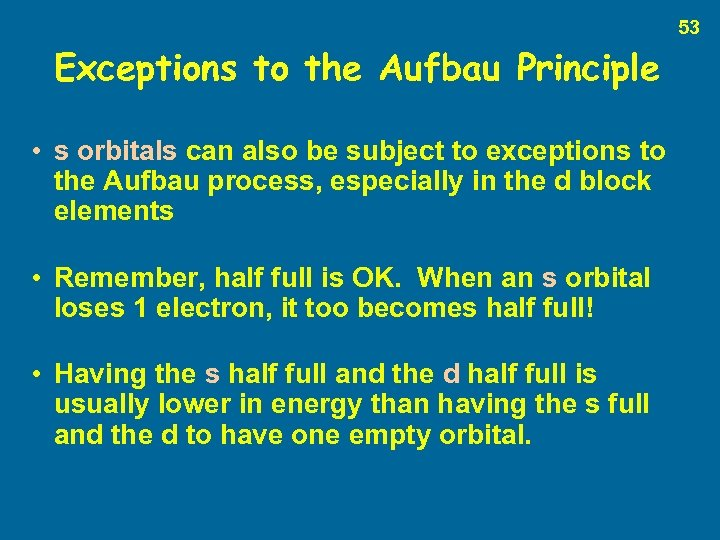53 Exceptions to the Aufbau Principle • s orbitals can also be subject to exceptions to the Aufbau process, especially in the d block elements • Remember, half full is OK. When an s orbital loses 1 electron, it too becomes half full! • Having the s half full and the d half full is usually lower in energy than having the s full and the d to have one empty orbital.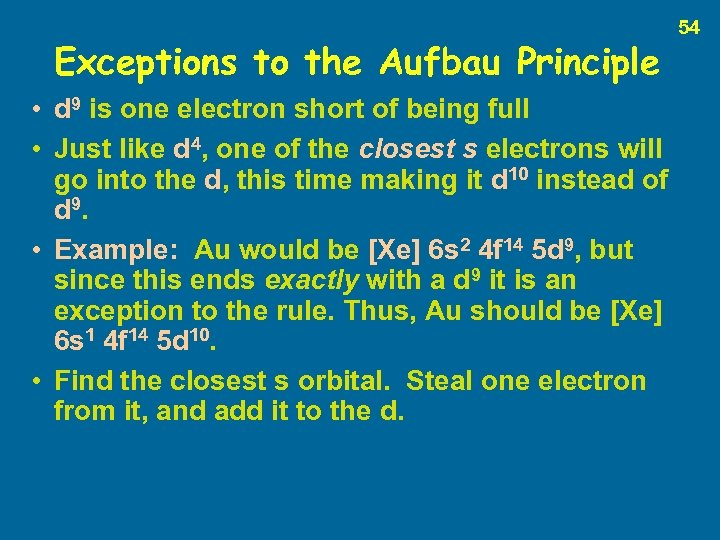Exceptions to the Aufbau Principle • d 9 is one electron short of being full • Just like d 4, one of the closest s electrons will go into the d, this time making it d 10 instead of d 9. • Example: Au would be [Xe] 6 s 2 4 f 14 5 d 9, but since this ends exactly with a d 9 it is an exception to the rule. Thus, Au should be [Xe] 6 s 1 4 f 14 5 d 10. • Find the closest s orbital. Steal one electron from it, and add it to the d. 54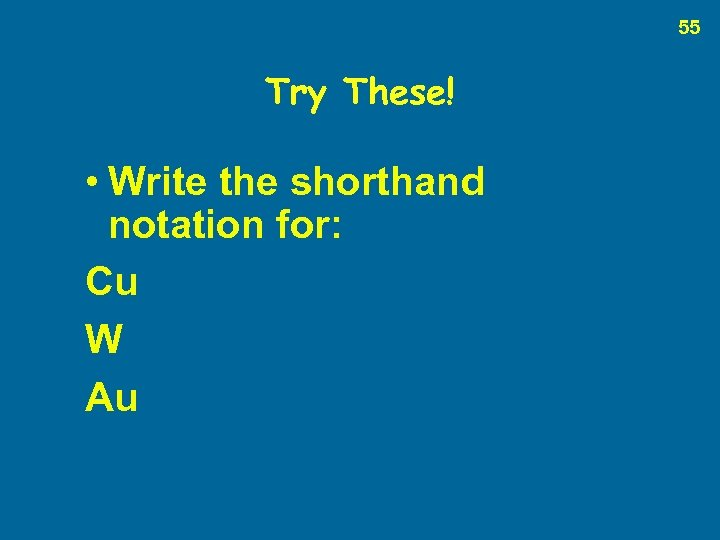55 Try These! • Write the shorthand notation for: Cu W Au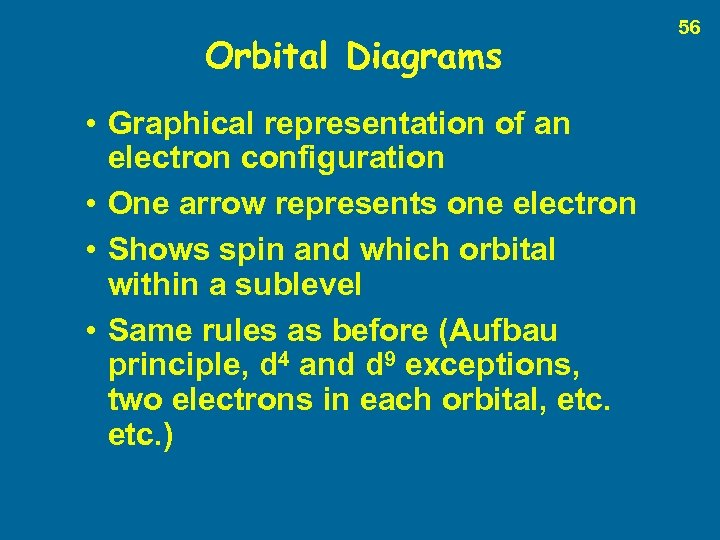Orbital Diagrams • Graphical representation of an electron configuration • One arrow represents one electron • Shows spin and which orbital within a sublevel • Same rules as before (Aufbau principle, d 4 and d 9 exceptions, two electrons in each orbital, etc. ) 56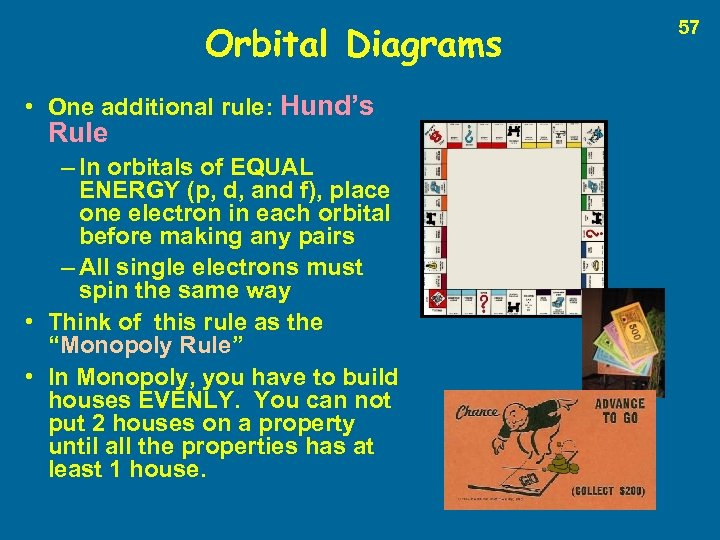Orbital Diagrams • One additional rule: Hund’s Rule – In orbitals of EQUAL ENERGY (p, d, and f), place one electron in each orbital before making any pairs – All single electrons must spin the same way • Think of this rule as the “Monopoly Rule” • In Monopoly, you have to build houses EVENLY. You can not put 2 houses on a property until all the properties has at least 1 house. 57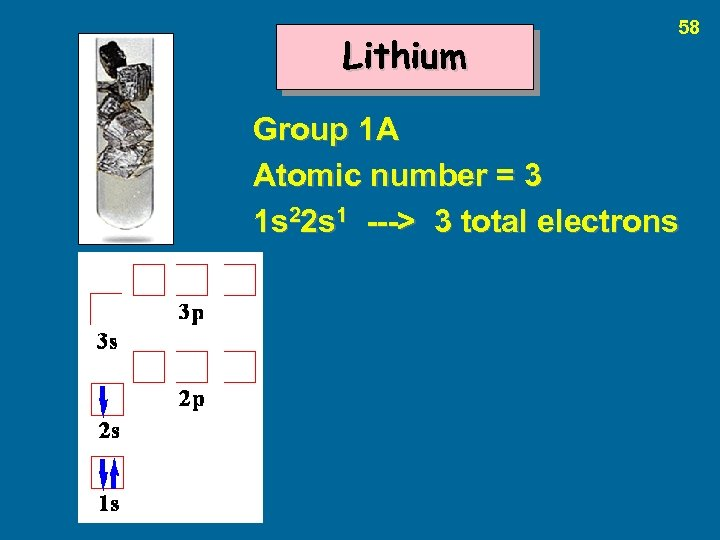Lithium 58 Group 1 A Atomic number = 3 1 s 22 s 1 ---> 3 total electrons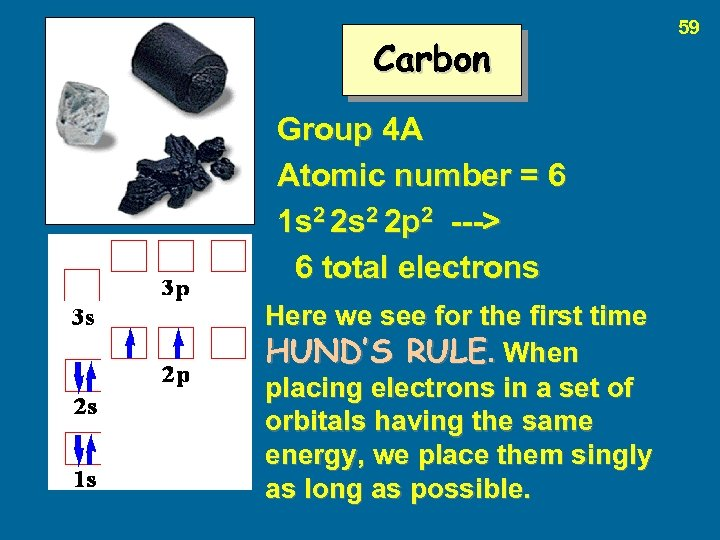Carbon Group 4 A Atomic number = 6 1 s 2 2 p 2 ---> 6 total electrons Here we see for the first time HUND’S RULE. When placing electrons in a set of orbitals having the same energy, we place them singly as long as possible. 59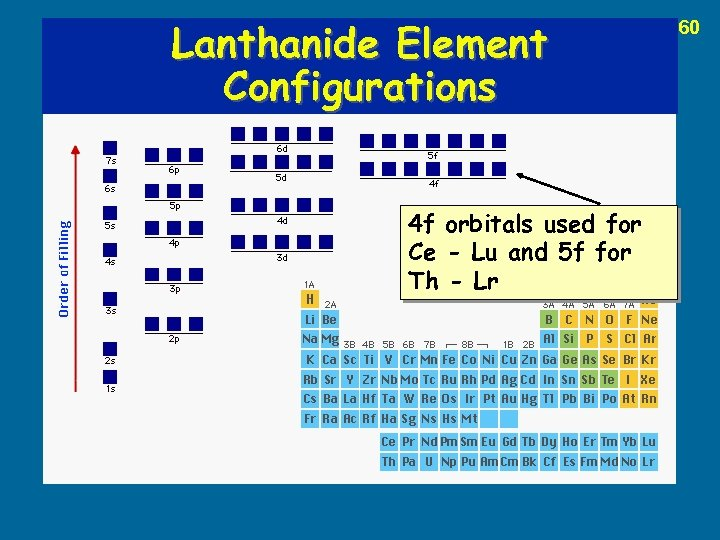Lanthanide Element Configurations 4 f orbitals used for Ce - Lu and 5 f for Th - Lr 60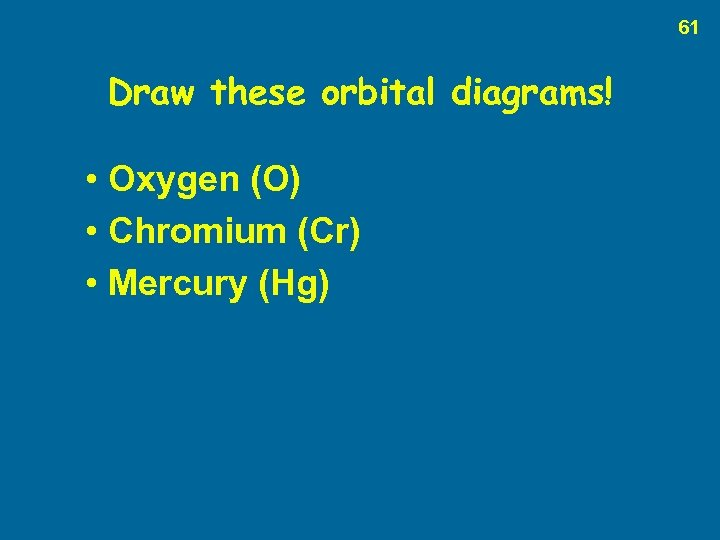61 Draw these orbital diagrams! • Oxygen (O) • Chromium (Cr) • Mercury (Hg)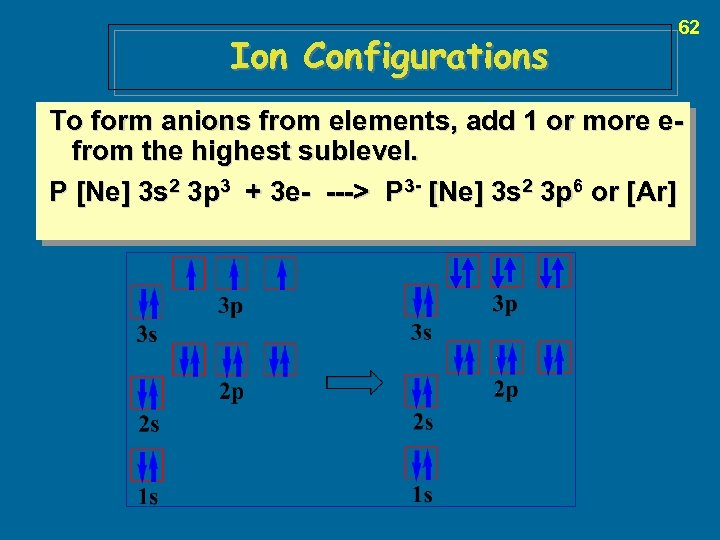Ion Configurations 62 To form anions from elements, add 1 or more efrom the highest sublevel. P [Ne] 3 s 2 3 p 3 + 3 e- ---> P 3 - [Ne] 3 s 2 3 p 6 or [Ar]63 The End !!!!!!!!!!